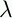September 22, 1999 Perl and the Lambda Calculus Slide #15

# Undefined Values

• Some expressions have no normal form:

• (x.(x x)x.(x x))

• Reduces to itself

• Now consider (y.P (x.(x x)x.(x x)))

• Could reduce immediately to P

• But if you try to evalute the argument first, you lose

• Is it hard to find normal forms? No.

• Theorem: Left-to-right evalaution always finds the normal form if one exists

• This is called normal-order evalutaion

• Note: Most languages use applicative order.

 NextCopyright © 1999 M-J. Dominus